NCERT Solutions: Introduction to Euclid’s Geometry (Exercise 5.1)

# Introduction to Euclid’s Geometry (Exercise 5.1) NCERT Solutions - Mathematics (Maths) Class 9

Q1: Which of the following statements are true and which are false? Give reasons for your answers.
(i) Only one line can pass through a single point.
(ii) There are an infinite number of lines which pass through two distinct points.
(iii) A terminated line can be produced indefinitely on both the sides
(iv) If two circles are equal, then their radii are equal.
(v) In Fig. 5.9, if AB = PQ and PQ = XY, then AB = XY.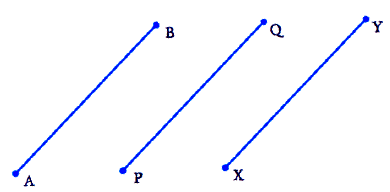Ans: (i) False

Reason: There can be infinite number of lines that can be drawn through a single point. Hence, the statement mentioned is False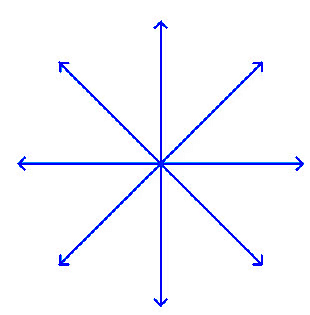(ii) False

Reason: Through two distinct points, there can be only one line that can be drawn. Hence, the statement mentioned is False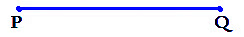(iii) True

Reason: A line that is terminated can be indefinitely produced on both sides as a line can be extended on both its sides infinitely. Hence, the statement mentioned is True.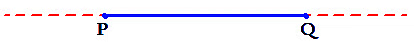(iv) True

Reason: The radii of two circles are equal when the two circles are equal. The circumference and the centre of both the circles coincide; and thus, the radius of the two circles should be equal. Hence, the statement mentioned is True.

(v) True

Reason: According to Euclid’s 1st axiom- “Things which are equal to the same thing are also equal to one another”. Hence, the statement mentioned is True.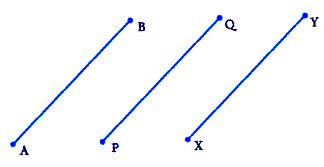Q2: Give a definition for each of the following terms. Are there other terms that need to be defined first? What are they, and how might you define them?
(i) parallel lines
(ii) perpendicular lines
(iii) line segment
(v) square
Ans:
Yes, we need to have an idea about the terms, point, line, ray, angle, plane, circle and quadrilateral, etc. before defining the required terms.
Point: A small dot made by a sharp pencil on the surface of paper gives an idea about a point.
It has no dimensions. It has only a position.
Line: A line is an idea that it should be straight and that it should extend indefinitely in both directions. It has no endpoints and has no definite length.

Note: In geometry, a line means “The line in its totality and not a portion of it. Whereas a physical example of a perfect line is not possible. A line extends indefinitely in both directions, so we cannot draw or show it wholly on paper. That is why we mark arrowheads on both ends, indicating that it extends indefinitely in both directions.

Ray: A part of a line that has only one endpoint and extends indefinitely in one direction. A ray has no definite length.
Angle: Two rays having a common endpoint form an angle.
Plane: A plane is a surface such that every point of the line joining any two points on it, lies on it.
Circle: A circle is the set of all those points in a given plane that are equidistant from a fixed point in the same plane. The fixed point is called the centre of the circle.
Definitions of the required terms are given below:
(i) Parallel Lines: Two lines ‘n’ and ‘m’ in a plane are said to be parallel if they have no common point and we write them as n || m.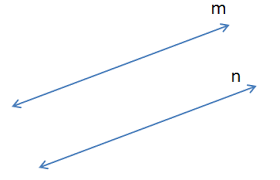Note: The distance between two parallel lines always remains the same.

(ii) Perpendicular Lines: Two lines ‘p’ and ‘q’ lying in the same plane are said to be perpendicular if they form a right angle and we write them as p ⊥ q.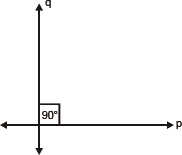(iii) Line Segment: A line segment is a part of the line having a definite length. It has two end-points.
In the figure, a line segment is shown having endpoints ‘A’ and ‘B’. It is written as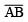or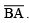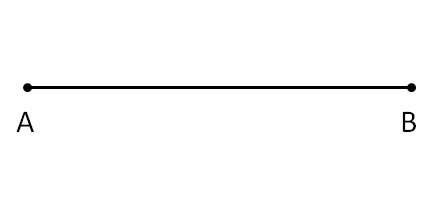(iv) Radius of a circle: The distance from the centre to a point on the circle is called the radius of the circle. In the figure, P is the centre and Q is a point on the circle, then PQ is the radius.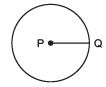(v) Square: A quadrilateral in which all the four angles are right angles and all the four sides are equal is called a square. In the figure, PQRS is a square.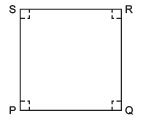Q3. Consider two ‘postulates’ given below:
(i) Given any two distinct points A and B, there exists a third point C which is in between A and B.
(ii) There exist at least three points that are not on the same line.
Do these postulates contain any undefined terms? Are these postulates consistent?
Do they follow Euclid’s postulates? Explain.
Ans: Yes, these postulates contain undefined terms. Undefined terms in the postulates are

• There are many points that lie in a plane. But, in the postulates given here, the position of point C is not given as to whether it lies on the line segment joining AB or not.
• On top of that, there is no information about whether the points are in the same plane or not.

And
Yes, these postulates are consistent when we deal with these two situations:

• Point C is lying on the line segment AB in between A and B.
• Point C does not lie on line segment AB.

Q4. If a point C lies between two points A and B such that AC = BC, then prove that AC =  (1/2)AB. Explain by drawing the figure.
Ans: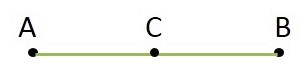Given that, AC = BC
Now, adding AC on both sides,
L.H.S.+AC = R.H.S.+AC
AC+AC = BC+AC
2AC = BC+AC
We know that BC+AC = AB (as it coincides with line segment AB)
∴ 2 AC = AB (If equals are added to equals, the wholes are equal.)
⇒ AC = (½)AB

Q5. In Question 4, point C is called a mid-point of line segment AB. Prove that every line segment has one and only one mid-point.
Ans: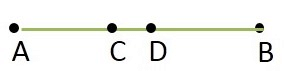Let AB be the line segment.
Assume that points P and Q are the two different midpoints of AB.
Now,
∴ P and Q are the midpoints of AB.
Therefore,
AP = PB and AQ = QB
also,
PB+AP = AB (as it coincides with line segment AB)
Similarly, QB+AQ = AB
Now,
Adding AP to the L.H.S. and R.H.S. of the equation AP = PB
We get AP+AP = PB+AP (If equals are added to equals, the wholes are equal.)
⇒ 2AP = AB — (i)
Similarly,
2 AQ = AB — (ii)
From (i) and (ii), Since R.H.S. are the same, we equate the L.H.S.
2 AP = 2 AQ (Things which are equal to the same thing are equal to one another.)
⇒ AP = AQ (Things which are double of the same things are equal to one another.)
Thus, we conclude that P and Q are the same points.
This contradicts our assumption that P and Q are two different midpoints of AB.
Thus, it is proved that every line segment has one and only one midpoint.
Hence, proved.

Q6. In the figure, given below, if AC = BD, then prove that AB = CD.
Ans: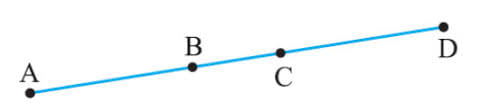It is given AC = BD
From the given figure, we get
AC = AB+BC
BD = BC+CD
⇒ AB+BC = BC+CD [AC = BD, given]
We know that, according to Euclid’s axiom, when equals are subtracted from equals, remainders are also equal.
Subtracting BC from the L.H.S. and R.H.S. of the equation AB+BC = BC+CD, we get
AB+BC-BC = BC+CD-BC
AB = CD
Hence, proved.

Q7. Why is Axiom 5, in the list of Euclid’s axioms, considered a ‘universal truth’? (Note that the question is not about the fifth postulate.)
Ans: Axiom 5: The whole is always greater than the part.
For Example: A cake. When it is whole or complete, assume that it measures 2 pounds but when a part from it is taken out and measured, its weight will be smaller than the previous measurement. So, the fifth axiom of Euclid is true for all the materials in the universe. Hence, Axiom 5, in the list of Euclid’s axioms, is considered a ‘universal truth’.

The document Introduction to Euclid’s Geometry (Exercise 5.1) NCERT Solutions | Mathematics (Maths) Class 9 is a part of the Class 9 Course Mathematics (Maths) Class 9.
All you need of Class 9 at this link: Class 9

## Mathematics (Maths) Class 9

42 videos|378 docs|65 tests

## FAQs on Introduction to Euclid’s Geometry (Exercise 5.1) NCERT Solutions - Mathematics (Maths) Class 9

 1. What is Euclid's Geometry?Ans. Euclid's Geometry is a branch of mathematics that deals with the study of plane and solid figures based on a set of axioms and theorems formulated by the ancient Greek mathematician Euclid. It is also known as Euclidean geometry and is the foundation of modern geometry.
 2. What are the axioms in Euclid's Geometry?Ans. Axioms in Euclid's Geometry are basic assumptions or statements that are considered to be true without proof. Euclid's axioms include statements like "a straight line can be drawn between any two points", "a straight line can be extended indefinitely", and "all right angles are congruent", among others.
 3. How is Euclid's Geometry different from non-Euclidean geometry?Ans. Euclid's Geometry is based on a set of axioms that assume a flat, two-dimensional space and follows the principles of Euclid's five postulates. Non-Euclidean geometry, on the other hand, explores geometries that do not adhere to these postulates and can exist in curved or higher-dimensional spaces.
 4. What are the main components of Euclid's Geometry?Ans. Euclid's Geometry consists of points, lines, and planes. Points are zero-dimensional entities that have no size or shape. Lines are one-dimensional objects that extend infinitely in both directions. Planes are two-dimensional surfaces that extend infinitely in all directions.
 5. How is Euclid's Geometry relevant in today's world?Ans. Euclid's Geometry is still relevant in various fields today, including architecture, engineering, computer graphics, and physics. It provides a framework for understanding and describing the properties and relationships of shapes and figures, helping us solve real-world problems and design structures efficiently.

## Mathematics (Maths) Class 9

42 videos|378 docs|65 testsExplore Courses for Class 9 examSignup to see your scores go up within 7 days! Learn & Practice with 1000+ FREE Notes, Videos & Tests.
10M+ students study on EduRev
Track your progress, build streaks, highlight & save important lessons and more!
Related Searches

,

,

,

,

,

,

,

,

,

,

,

,

,

,

,

,

,

,

,

,

,

;